# 社区划分-PageRank算法的解析与Python实现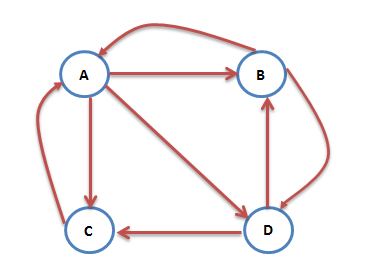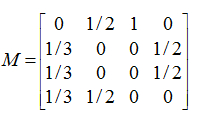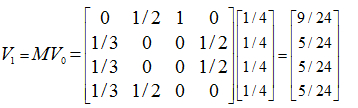注意矩阵M中M[i][j]不为0表示用一个链接从j指向i，M的第一行乘以V0，表示累加所有网页到网页A的概率即得到9/24。得到了V1后，再用V1去右乘M得到V2，一直下去，最终V会收敛，即Vn=MV(n-1)，上面的图示例，不断的迭代，最终V=[3/9,2/9,2/9,2/9]‘：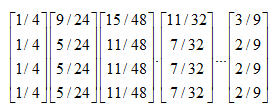• 图是强连通的，即从任意网页可以到达其他任意网页：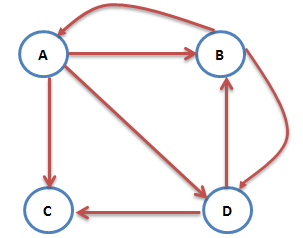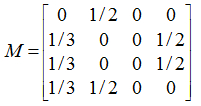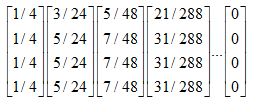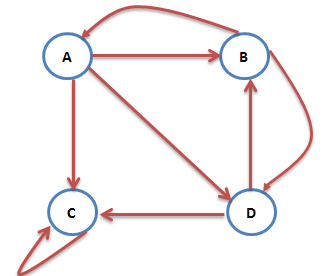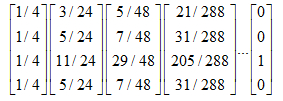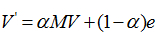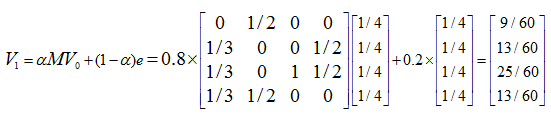from numpy import *

a = array([[0,1,1,0],
[1,0,0,1],
[1,0,0,1],
[1,1,0,0]],dtype = float)  #dtype指定为float

def graphMove(a):   #构造转移矩阵
b = transpose(a)  #b为a的转置矩阵
c = zeros((a.shape),dtype = float)
for i in range(a.shape):
for j in range(a.shape):
c[i][j] = a[i][j] / (b[j].sum())  #完成初始化分配
#print c,"\n===================================================="
return c

def firstPr(c):   #pr值得初始化
pr = zeros((c.shape,1),dtype = float)  #构造一个存放pr值得矩阵
for i in range(c.shape):
pr[i] = float(1)/c.shape
#print pr,"\n==================================================="
return pr

def pageRank(p,m,v):  #计算pageRank值
while((v == p*dot(m,v) + (1-p)*v).all()==False):  #判断pr矩阵是否收敛,(v == p*dot(m,v) + (1-p)*v).all()判断前后的pr矩阵是否相等，若相等则停止循环
#print v
v = p*dot(m,v) + (1-p)*v
#print (v == p*dot(m,v) + (1-p)*v).all()
return v

if __name__=="__main__":
M = graphMove(a)
pr = firstPr(M)
p = 0.8           #引入浏览当前网页的概率为p,假设p=0.8
print pageRank(p,M,pr)  # 计算pr值【技术服务】，详情点击查看：https://mp.weixin.qq.com/s/PtX9ukKRBmazAWARprGIAg©️2019 CSDN 皮肤主题: 大白 设计师: CSDN官方博客